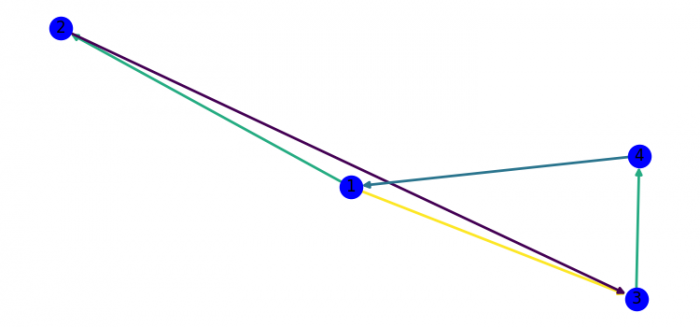# Colouring the edges by weight in networkx (Matplotlib)

MatplotlibPythonData Visualization

To color the edges by weight in networkx, we can take the following steps −

• Set the figure size and adjust the padding between and around the subplots.
• Initialize a graph with edges, name, or graph attributes.
• Add nodes to the current graph.
• Add edges to the current graph's nodes.
• Iterate the given graph's edges and set some weight to them.
• Draw current graphs with weights for edge color.
• To display the figure, use show() method.

## Example

import random as rd
import matplotlib.pylab as plt
import networkx as nx

plt.rcParams["figure.figsize"] = [7.50, 3.50]
plt.rcParams["figure.autolayout"] = True

G = nx.DiGraph()
plt.show()